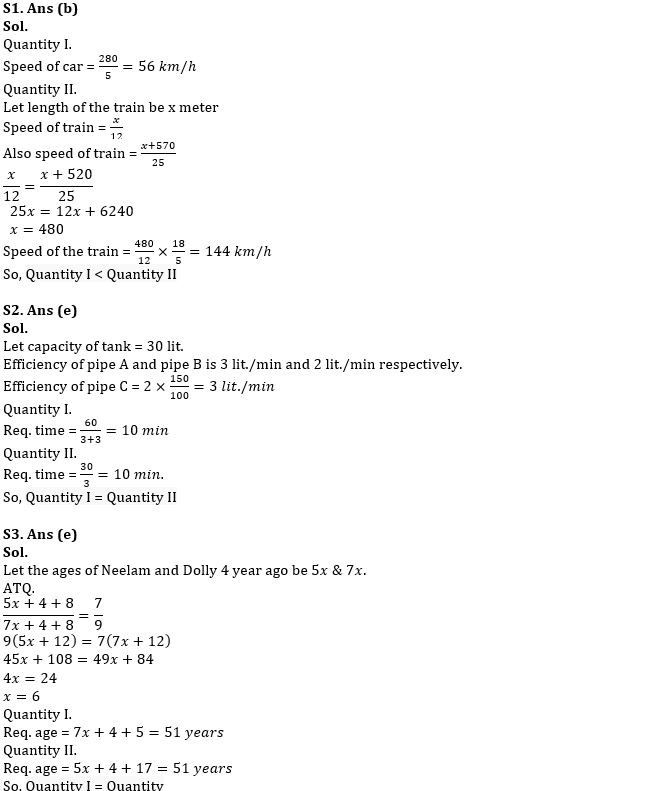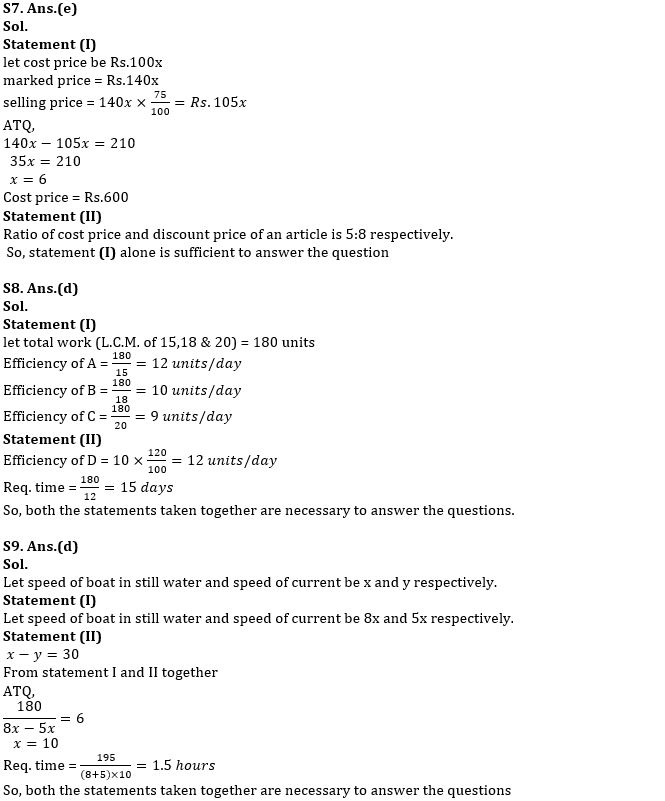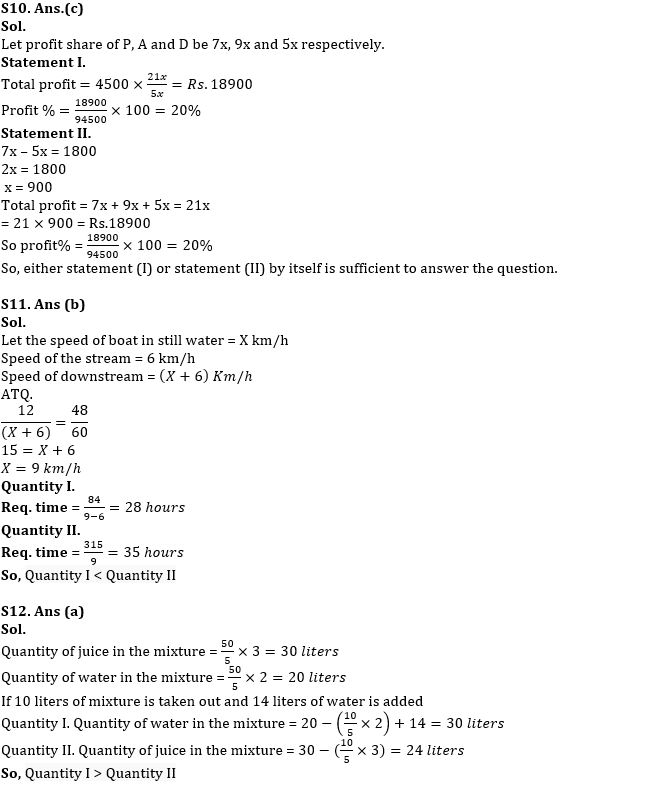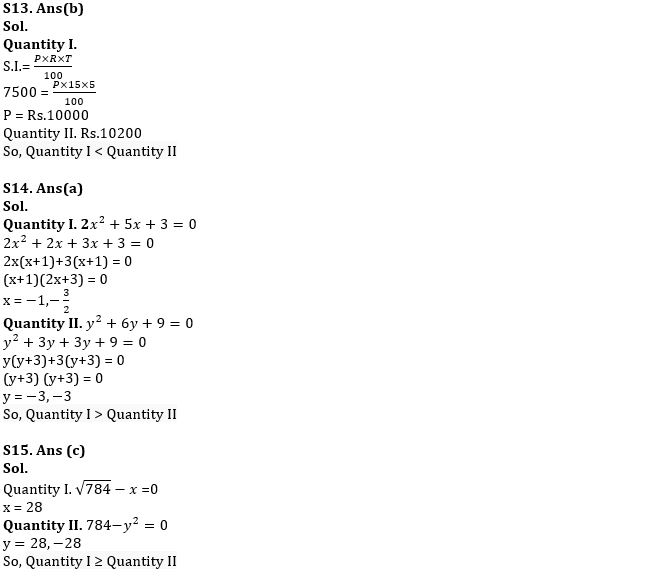Latest Banking jobs   »

# Quantitative Aptitude Quiz For IBPS Clerk/PO Prelims 2022- 13th September

Directions (1–5): In the given questions, two quantities are given, one as ‘Quantity I’ and another as ‘Quantity II’. You have to determine relationship between two quantities and choose the appropriate option
(a) Quantity I > Quantity II
(b) Quantity I < Quantity II
(c) Quantity I ≥ Quantity II
(d) Quantity I ≤ Quantity II
(e) Quantity I = Quantity II or no relation

Q1. Quantity I. A car can travel 280 km in 5 hours. Find the speed of car. (in kmph)
Quantity II. A train can cross a pole and bridge having a length of 520 m in 12 secs and 25 secs respectively. Find the speed of the train (in kmph).

Q2. Pipe A and pipe B can fill the tank in 10 min and 15 min respectively. Efficiency of pipe C is 50% more than that of B.
Quantity I. Find the time taken (in min) by pipe C and A when both opened simultaneously to fill double the capacity of the tank.
Quantity II. Find the time taken (in min) by pipe C to fill the tank alone.

Q3. 4 years ago, the ratio of the ages of Neelam and Dolly is 5: 7. Eight years hence the ratio of their ages will be 7:9
Quantity I. Find the age of Dolly 5 years hence.
Quantity II. Find the age of Neelam 17 years hence.

Q4. Quantity I. x; 12x²-x-1=0
Quantity II. y; 20y²-41y+20=0

Q5. The ratio of speed of boat in still water to the speed of stream is 11: 5. A boat goes 24 km in 45 minutes downstream.
Quantity I. Find the time taken (in min) by boat to cover the distance of 160 km downstream?
Quantity II. Find the time taken (in min) by boat to cover the distance of 48 km upstream?

Directions (6-10): The following questions are accompanied by two statements i.e. statement (I) and statement (II). You have to determine which statements(s) is/are sufficient/necessary to answer the questions.
(a) Neither statement (I) nor statement (II) by itself is sufficient to answer the question.
(b) Statement (II) alone is sufficient to answer the question but statement (I) alone is not sufficient to answer the question.
(c) Either statement (I) or statement (II) by itself is sufficient to answer the question.
(d) Both the statements taken together are necessary to answer the questions, but neither of the statements alone is sufficient to answer the question.
(e) Statement (I) alone is sufficient to answer the question but statement (II) alone is not sufficient to answer the questions

Q6. Find the area of rectangle.
Statement (I) Area of square is 64 cm².
Statement (II) Side of square is half the length of rectangle. Ratio of breadth to length of rectangle is 3:4 respectively.

Q7. Find the cost price of article.
Statement (I) Shopkeeper marked the article 40% above the cost price and allow 25% discount on marked price. Difference between selling price and marked price of the article is Rs.210.
Statement (II) Ratio of cost price and discount price of the article is 5:8 respectively.

Q8. Find the time taken by D to complete the whole work.
Statement (I) A, B and C can complete the work in 15 days, 18 days and 20 days respectively.
Statement (II) Efficiency of D is 20% more than that of B.

Q9. Find the time taken by boat to cover 195 km in downstream.
Statement (I) Ratio of speed of boat in still water to current is 8:5 respectively.
Statement (II) The boat can travel 180 km in upstream in 6 hours.

Q10. P, A and D invested Rs.94500 in a business in the ratio 7: 9: 5 respectively for a year. What is the profit % earned by them after a year?
Statement (I) D got Rs. 4500 as his share of profit.
Statement (II) The difference in profit earned by P and D is Rs.1800.

Directions (11–15): In the given questions, two quantities are given, one as ‘Quantity I’ and another as ‘Quantity II’. You have to determine relationship between two quantities and choose the appropriate option
(a) Quantity I > Quantity II
(b) Quantity I < Quantity II
(c) Quantity I ≥ Quantity II
(d) Quantity I ≤ Quantity II
(e) Quantity I = Quantity II or no relation

Q11. A boat goes 12 km downstream in 48 minutes and the speed of the stream is 6 km/h.
Quantity I. Find the time taken (in hours) by boat to cover 84 km upstream.
Quantity II. Find the time taken (in hours) by boat to cover 315 km in still water.

Q12. The vessel has 50 liters of mixture of juice and water in the ratio of 3:2 respectively. 10 liters of mixture is taken out and 14 liters of water is added in the mixture.
Quantity I. Find the final quantity (in liter) of water in the mixture.
Quantity II. Find the final quantity (in liter) of juice in the mixture.

Q13. Quantity I. The simple interest on certain sum of money for 5 years at 15% p.a. is Rs.7500. Find the sum (in Rs.).
Quantity II. Rs.10200

Q14. Quantity I. x; 2x²+5x+3=0
Quantity II. y; y²+6y+9=0

Q15. Quantity I. x; √784-x =0
Quantity II. y; 784-y²=0

Solutions#### Congratulations!Download Hindu Review of October 2021: Free PDF